##### PostgreSQL DIV() Function With Practical Examples

PostgreSQL offers numerous built-in mathematical functions such as COUNT(), AVG(), MAX(), DIV(), etc. All these functions are responsible for performing different functionalities, such as AVG() function calculates the average, MAX() function finds the maximum number, and so on. Similarly, the DIV() function is responsible for performing integer division.

This blog post will provide a thorough overview of the DIV() function via practical examples. So, let’s get started.

How to Use the DIV() Function in Postgres?

PostgreSQL provides a built-in mathematical function named DIV() that takes two numeric values as arguments, performs division on them, and retrieves the resultant integer. PostgreSQL's DIV() function has the following syntax:

`DIV(arg_1, arg_2);`

Here, arg_1 represents the dividend, while arg_2 represents the divisor. The DIV() function will divide the dividend “arg_1” with the divisor “arg_2” and retrieve a resultant integer(quotient).

Let’s understand the DIV() function via practical examples.

Example 1: Pass Two Positive Values to DIV() Function

The below snippet demonstrates the working of the DIV() function:

`SELECT DIV(12, 8);`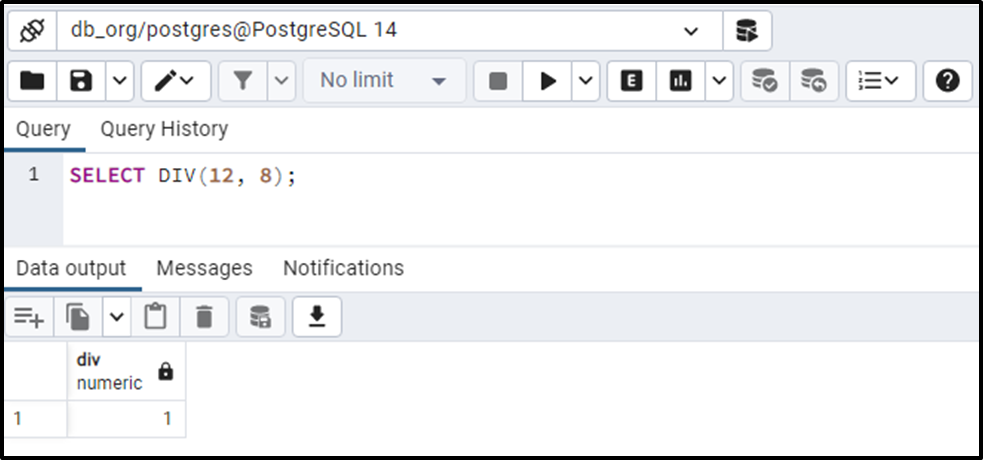The output proves that the DIV() function divides the dividend “12” with the divisor “8” and retrieves the quotient “1”.

Example 2: Pass Two Negative Values to DIV() Function

Let’s consider the following statement to see how the DIV() function deals with negative values:

`SELECT DIV(-120, -7);`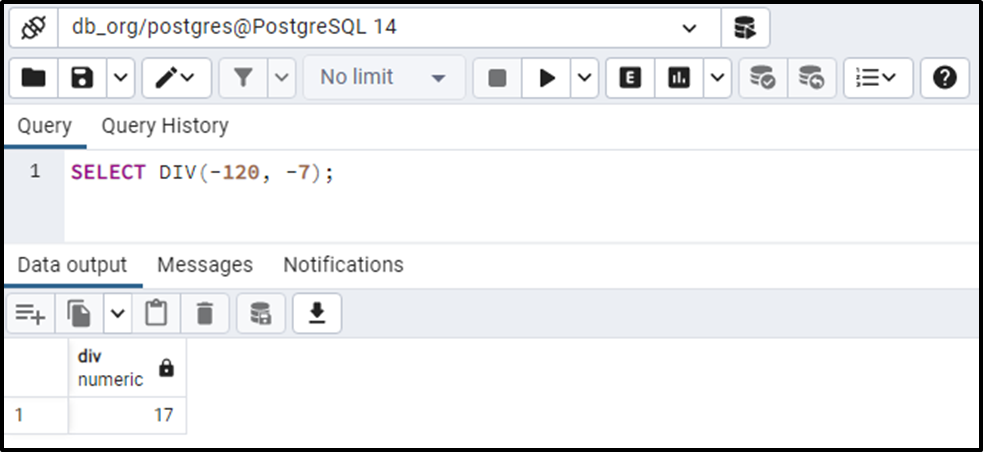The output shows that the DIV() function performs the division on the given numbers and retrieves a numeric value.

Example 3: Pass One Positive and One Negative Value to DIV() Function

Let’s pass a negative dividend and positive devisor and see how the DIV() function works:

`SELECT DIV(-120, 7);`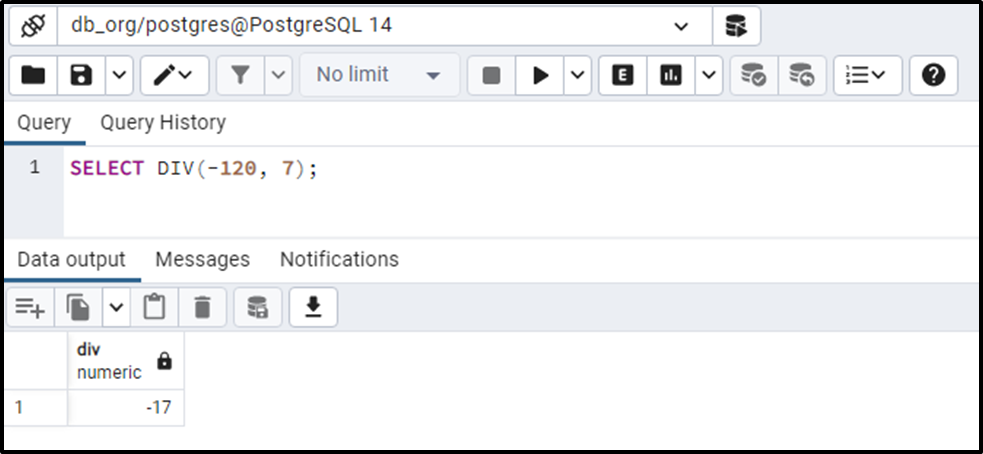The output shows that the DIV() function retrieves the appropriate result.

Example 4: Pass Fractional Values to DIV() Function

Let’s learn how does the DIV() function work with the fractional values:

`SELECT DIV(123.43, 14.54);`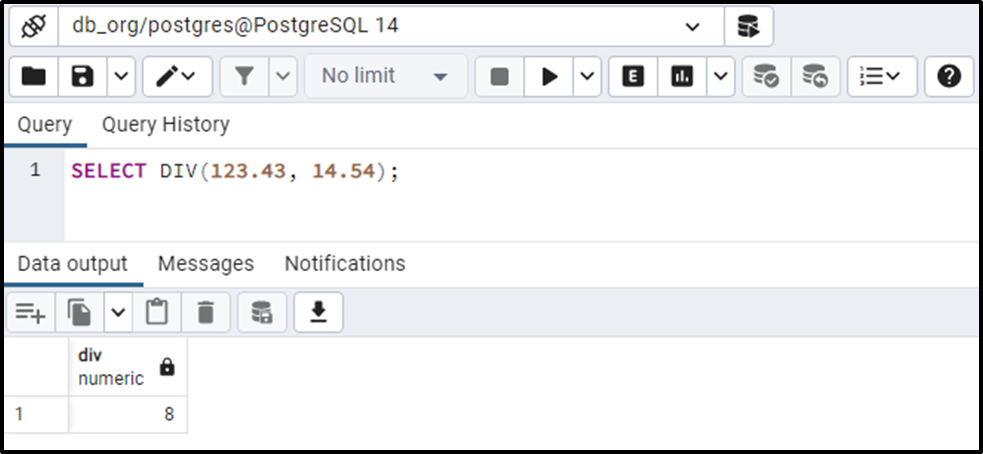The output shows that the DIV() function performs division on the given fractional values and retrieves an integer value.

Example 5: How to Use DIV() Function on Table’s Data?

Let’s create a table div_example that consists of two columns: num1, and num2:

```CREATE TABLE div_example (
num1 NUMERIC,
num2 NUMERIC
);```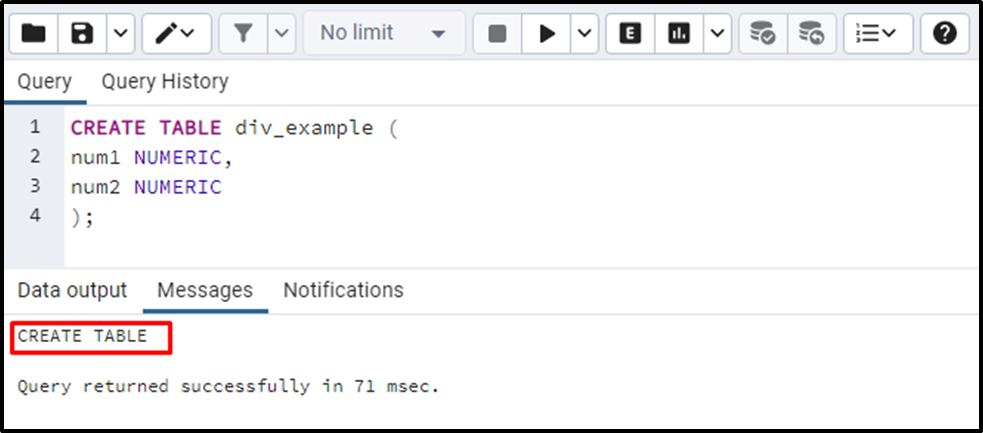A table named “div_example” has been created. Let’s insert some data into the “div_example” table:

```INSERT INTO div_example(num1, num2)
VALUES(1920, 11),
('Seth', 890, 6),
('Mike', 675, 3),
('Joseph', 107, 15);```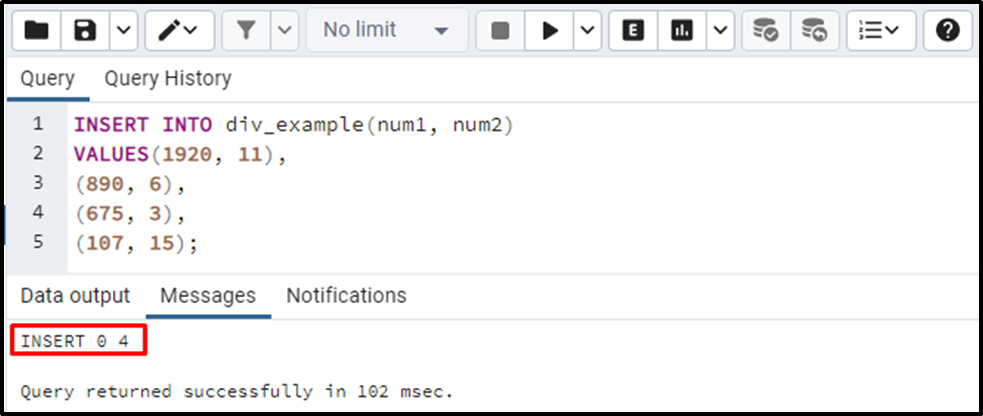Four records have been inserted into the div_example table. Let’s implement the DIV() function on the div_example table to perform the division on the num1 and num2 columns:

```SELECT DIV(num1, num2)
FROM div_example;```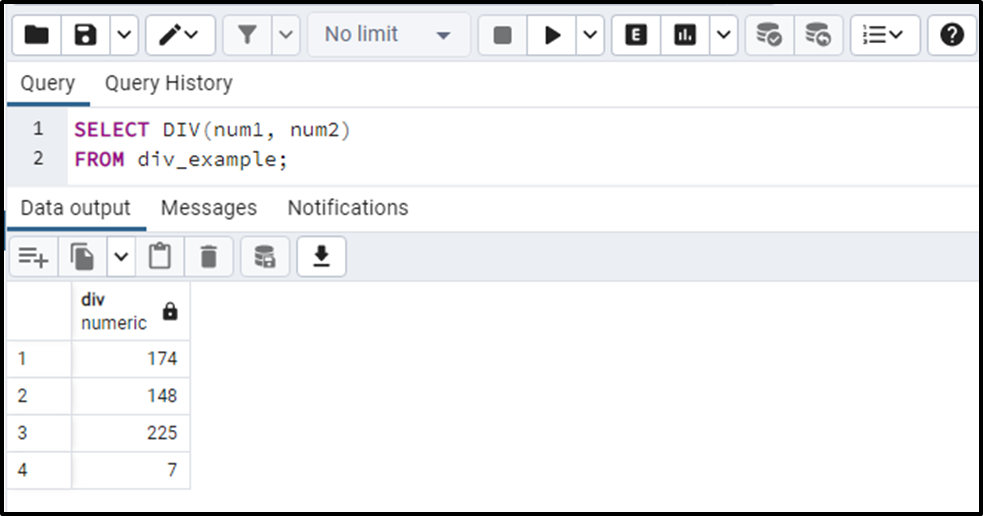The output authenticates the working of the DIV() function as it retrieves the appropriate results.

That’s it from this Postgres guide.

Conclusion

PostgreSQL provides a built-in DIV() function that takes two numeric values as arguments, performs division on them, and retrieves the resultant integer. The DIV() function accepts any positive values, negative values, fractional/floating point values, etc. as arguments and retrieves an integer value. This blog post explained different use cases of the DIV() function via practical examples.